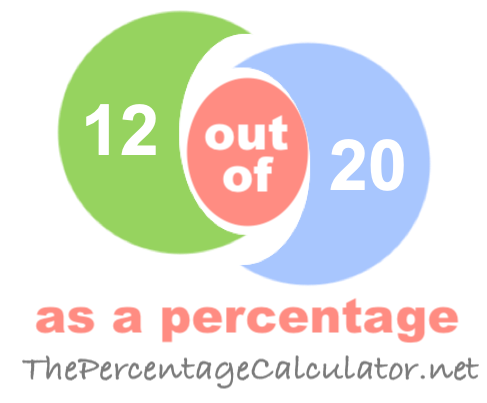What is 12 out of 20 as a percentage?When you ask "What is 12 out of 20?" you want to know what percent 12 is out of 20.

Here are step-by-step instructions showing you how we calculated 12 out of 20 as a percentage:

The first step is to divide 12 by 20 to get the answer in decimal form:

12 / 20 = 0.6000

Then, we multiplied the answer from the first step by one hundred to get the answer as a percentage:

0.6000 * 100 = 60.00%

We can prove that the answer is correct by taking 60.00 percent of 20 to get 12:

(20 x 60.00)/100 = 12

Note that our calculator rounds the answers up to two decimals if necessary. Once again, the answer is as follows:

60.00%

Out of as a Percentage Calculator
To solve another problem, please submit it below:

out of

What is 12 out of 21 as a percentage?
The solution to "What is 12 out of 20 as a percentage?" is not the only answer we have. Go here for the next solution on our list.